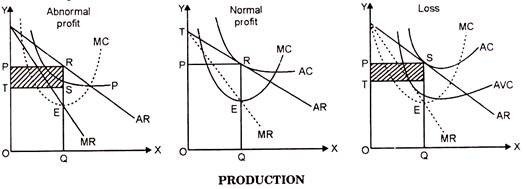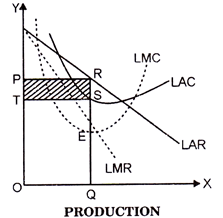# Price Determination of Firm and industry under Monopoly

A monopoly refers to when a company and its product offerings dominate one sector or industry. Monopolies can be considered an extreme result of free-market capitalism in that absent any restriction or restraints, a single company or group becomes large enough to own all or nearly all of the market (goods, supplies, commodities, infrastructure, and assets) for a particular type of product or service. The term monopoly is often used to describe an entity that has total or near-total control of a market.

Monopolies typically have an unfair advantage over their competition since they are either the only provider of a product or control most of the market share or customers for their product. Although monopolies might differ from industry-to-industry, they tend to share similar characteristics that include:

• High or no barriers to entry: Competitors are not able to enter the market, and the monopoly can easily prevent competition from developing their foothold in an industry by acquiring the competition.
• Single seller: There is only one seller in the market, meaning the company becomes the same as the industry it serves.
• Price maker: The company that operates the monopoly decides the price of the product that it will sell without any competition keeping their prices in check. As a result, monopolies can raise prices at will.
• Economies of scale: A monopoly often can produce at a lower cost than smaller companies. Monopolies can buy huge quantities of inventory, for example, usually a volume discount. As a result, a monopoly can lower its prices so much that smaller competitors can’t survive. Essentially, monopolies can engage in price wars due to their scale of their manufacturing and distribution networks such as warehousing and shipping, that can be done at lower costs than any of the competitors in the industry.

Determination of Price Under Monopoly

Under Monopoly every seller wants to earn maximum Profit.

This fact Prof. Marshall has stated that monopolist wants to earn “Maximum Monopoly Gain” by selling his goods.

This thing Mrs. Robinson has stated as Net Monopoly Revenue.

Now, the important question arises that how monopolist should fix his price, so that he may earn maximum profit? On this point two economists written above are of this opinion the price determination under monopoly condition is similar to those of perfect competition.

The only difference is that in perfect competition the average revenue curve and marginal revenue curve are same and parallel to X-axis where as in Monopoly these curves are downwards sloping curves. The Monopolist behaves like a firm. His aim is maximization of profits and if there are losses, then minimization of losses. The profits are maximized when marginal cost is equal to marginal revenue. The losses are minimum where marginal cost is equal to marginal revenue but afterwards marginal cost must be rising.

A Monopolist being the only producer and seller of that commodity can determine its price and the quantity of its production or supply. He cannot do both the things simultaneously. Either he fixes the price and leaves the output to be determined by the consumer demand at that price or he can fix the output to be produced and leave the price to be determined by the consumers’ demand for his product. But it is a common experience that he leaves the price to the market mechanism and determines the volume of output. Under no circumstances, he will be ready to bear losses.

If, in a short period, the cost of production of a commodity is zero, he will go on producing it to the extent or so long the marginal revenue from the sale of that commodity does not fall to zero. As soon as the marginal reserve is zero he will not increase its supply.

Some economists think that, in a short period, three different situations may arise before the monopolist:

(i) When the monopolist earns abnormal profits,

(ii) When he gets only normal profits, and

(iii) When he suffers losses.

The explanation and diagrams of these situations are given below:On the point E the firm is in equilibrium when MC = MR. Thereafter MC curve starts to rise. Under the condition, OP is the price and OQ is the ‘total production’ of the commodity so determined. In order to calculate profits or losses, we will have to measure the difference between AR and AC. If AR > AC, the difference between the two is profit per unit and by multiply it with total number of units produced we can get total profit.

In the first figure RQ = OP is the price, TO is the cost of production per unit. Thus, RS =PT is unit for profit. On the OQ quantity of production, total profit is PTSR shaded area which is abnormal profit. In the second figure RQ = OP is the determined price and RQ is the average cost. Under this condition, there will be only normal profit.

In the figure three also price per unit is RQ = OP but cost per unit is SQ. Thus, SR (TP) is loss per unit. As a result TPRS shaded area will be the total loss. But this loss is only short period phenomenon. In the long period, this loss will disappear, under that condition and situation, only profit will be earned.

Determination of Price in the Long Period

In the long period the monopolist introduces changes in his equipment’s and techniques of production. During this period in order to gain excess profit, he will change efficiency and capacity of his resources according to his need. But the determination of the quantity of production follows, the same line as under short period.

This is clear from the following figure:In this figure LMC and LMR intersect each other at the point E and after that LMC goes on rising. Thus OQ production is determined and OP is the price. But average cost is SQ. So profit per unit is RS and at OQ output the total profit is PTSR.

Under Price Competition AR =MR, where-as under Monopoly MR <AR.

Under perfect competition price is determined by the interaction of total demand and supply. This price is acceptable to all the firms in the industry. No firm can change this price. So, average revenue and marginal revenue, at every level of production, will be constant and equal. Their curves are parallel to X-axis.

Under Monopoly, to sell every additional unit of the commodity price will have to be lower. In this way, with the sale of every additional unit, average and marginal income goes on falling. But the decrease in average revenue is relatively less sharp than the decrease in marginal revenue, It is because marginal revenue is limited to one unit, whereas in case of average revenue, the decrease price is divided by the number of units. Therefore, the fall in average revenue has relatively less slope. That is the reason why marginal revenue is less than average revenue.#### intactone

View all posts by intactone →
error: Content is protected !!